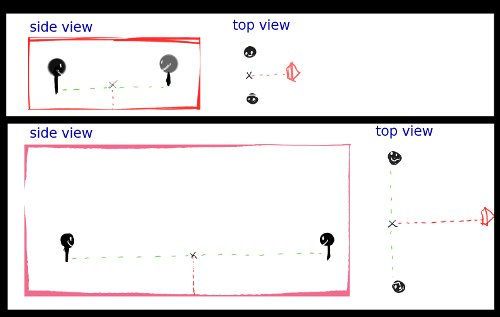# Keep 2 objects in the camera view

Hi,

I would like to understand how to achieve that effect (see image).

Explanations
When 2 objects or characters are close, the camera is close.
When the 2 objects are moving away, independently, the camera tries to keep both of them in the frame.

I’ve tried doing it by making my camera track the location between the two objects and align on an axis (it depends on the situation).
From that, I can move my camera away from that empty (but still track it) on an axis so that the camera view is larger.

The problem is : how can I calculate the good distance so that both objects are inside the “frustrum”?

I’d like to be able to know : if my camera is x units from the empty, and the camera is tracking the empty, one or both objects are outside the view.

Should I use (and study) maths for that?

Thank you!

### AttachmentsIf I remember trigonometry right here is how to do it:
The objects We have the objects A and B, and the camera C. Between the objects we have the spot D. A-C-D forms a triangle with a right angle at D, therefore the the tangent of the angle © at C is the distance A-D (lets call it a) divided by the distance A-C (lets call it b). tan© = a/b which is equivalent with b = a/tan©.

The distance the camera has to be from the empty is: the distance between the empty and one of the objects divided by tangent of the angle you want. I’m not sure what the camera view angle is. But import math and give it a try.

isnt it 45 degrees?? and if it is, the distance between your empty and your camera should be equal to (or a little more than) the greater of the two distances of the characters from the empty…

Hi,
first of all thanks for the help.

I’m still trying to get it to work.
I’d like to be able to do it by myself, but can somebody tell me how I can do the following :
let’s say there are a plane and a sphere in my scene.
I’d like to put my sphere 10 units from the plane, following the plane’s Y axis.

How can I do that?

Haha Cray I love the explanation drawings you always do. <3

There are many ways to do it. The most efficient I think would be to use Tales, which means simply keeping the ratio of the char distance / camera distance the same. Store the value of the distance between the characters and the distance from the empty to the camera, save the ratio on initialization, then apply that ration every game tick.

Parent the camera to the Empty, so it can move in it’s local space to get closer/further. On the camera, have a script like this and tell the controller to run the “loop” function every gametick:

``````import GameLogic
cont = GameLogic.getCurrentController()
own = cont.owner
scene = GameLogic.getCurrentScene()

char1 = scene.objects("OBChar1") # put char1 name here
char2 = scene.objects("OBChar2") # put char2 name here

char_distance = char1.getDistanceTo(char2)
camera_distance = own.localPosition # &lt;- not sure if this is the right axis, change if needed
ratio = camera_distance/char_distance

def loop():
char_distance = char1.getDistanceTo(char2)
camera_distance = ratio*char_distance/
p = camera.localPosition
p = camera_distance # again make sure this is the right axis
camera.localPosition = p

``````

You have to change the object names the put the right axis to make the camera move torward it’s parent (the empty). If there is no silly syntax error, then this should work.

• parent sphere to plane so they always have same orientation and move the sphere 10 units on it’s Y axis

• or copy the rotation from plane to sphere with sphere.worldOrientation = plane.worldOrientation ;Then move the sphere 10 units on it’s Y axis.

• or get a vector of the plane orientation relative to Y with v = plane.getAxisVect([0,1,0]) ; multiply each axis of the vector by 10 with v *= 10; v *= 10; v *= 10 ; then sum that vector to the sphere with p = sphere.worldPosition ; add v…v to p…p then apply with sphere.worldPosition = p

• or import mathutils and do the same as before with less lines: v = Vector(plane.getAxisVect([0,1,0]) ; p = Vector(sphere.worldPosition) ; p += v*10 ; sphere.worldPosition = p

Thank you, I mixed a bit of all of that and got the result I was expecting.
With your first script, I had the problem of the camera position keeping updated (+10, +10, +10 etc…) and flying through the scene. Maybe that was due to my scripts architecture.

Haha Cray I love the explanation drawings you always do. <3

It’s easier for me to explain that wayI know that good explanations of the issue = good and fast answers
and I enjoy doing it.

Last question (maybe not worth opening a new thread?) :
How to be sure that an initialization script is exectuded before the others?
I use the Controller’s star but even if I do it, the script is the last one to be executed (I use print to check).

Is the script priority connected to the position of an object in a hierarchy? (parent, child)
I open a new thread, the answers to that question might be useful for somebody